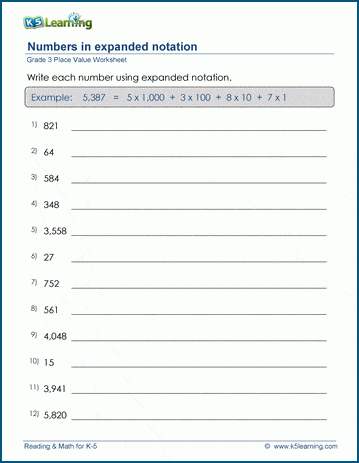# How to Write Numbers using Expanded Notation

Expanded notation is a way to represent a number as the sum of its individual place values. Each digit in a number is expanded to show its place value, making it easier to understand the number's composition.

Here's how to write numbers using expanded notation.

Let's demonstrate this with an example: 3,456.

Identify the place values:

In our example, we have thousands, hundreds, tens, and ones places. So, the place values are 1000, 100, 10, and 1, respectively.

Write the digits in the number in terms of their place values:

The digit in the thousands place (3) is expanded as 3 x 1000.

The digit in the hundreds place (4) is expanded as 4 x 100.

The digit in the tens place (5) is expanded as 5 x 10.

The digit in the ones place (6) is expanded as 6 x 1.

Combine the expanded forms to express the number:

3,456 in expanded form is:

3 x 1000 + 4 x 100 + 5 x 10 + 6 x 1

Now, you can calculate the products and add them together:

3 x 1000 = 3000

4 x 100 = 400

5 x 10 = 50

6 x 1 = 6

So, 3,456 in expanded form is 3000 + 400 + 50 + 6, which equals 3,456.

## Expanded notation worksheets

In our grade 3 place value section we have a set of worksheets for students to practice writing numbers in expanded notation.Become a Member

This content is available to members only.